# 1.10: Organic Chemistry (Exercises)

•• OpenStax
• OpenStax
$$\newcommand{\vecs}{\overset { \rightharpoonup} {\mathbf{#1}} }$$ $$\newcommand{\vecd}{\overset{-\!-\!\rightharpoonup}{\vphantom{a}\smash {#1}}}$$$$\newcommand{\id}{\mathrm{id}}$$ $$\newcommand{\Span}{\mathrm{span}}$$ $$\newcommand{\kernel}{\mathrm{null}\,}$$ $$\newcommand{\range}{\mathrm{range}\,}$$ $$\newcommand{\RealPart}{\mathrm{Re}}$$ $$\newcommand{\ImaginaryPart}{\mathrm{Im}}$$ $$\newcommand{\Argument}{\mathrm{Arg}}$$ $$\newcommand{\norm}{\| #1 \|}$$ $$\newcommand{\inner}{\langle #1, #2 \rangle}$$ $$\newcommand{\Span}{\mathrm{span}}$$ $$\newcommand{\id}{\mathrm{id}}$$ $$\newcommand{\Span}{\mathrm{span}}$$ $$\newcommand{\kernel}{\mathrm{null}\,}$$ $$\newcommand{\range}{\mathrm{range}\,}$$ $$\newcommand{\RealPart}{\mathrm{Re}}$$ $$\newcommand{\ImaginaryPart}{\mathrm{Im}}$$ $$\newcommand{\Argument}{\mathrm{Arg}}$$ $$\newcommand{\norm}{\| #1 \|}$$ $$\newcommand{\inner}{\langle #1, #2 \rangle}$$ $$\newcommand{\Span}{\mathrm{span}}$$$$\newcommand{\AA}{\unicode[.8,0]{x212B}}$$

1. Write the chemical formula and Lewis structure of the following, each of which contains five carbon atoms:

1. an alkane
2. an alkene
3. an alkyne
There are several sets of answers; one is:

(a) C5H12(b) C5H10(c) C5H82. What is the difference between the hybridization of carbon atoms’ valence orbitals in saturated and unsaturated hydrocarbons?

3. On a microscopic level, how does the reaction of bromine with a saturated hydrocarbon differ from its reaction with an unsaturated hydrocarbon? How are they similar?

Both reactions result in bromine being incorporated into the structure of the product. The difference is the way in which that incorporation takes place. In the saturated hydrocarbon, an existing C–H bond is broken, and a bond between the C and the Br can then be formed. In the unsaturated hydrocarbon, the only bond broken in the hydrocarbon is the π bond whose electrons can be used to form a bond to one of the bromine atoms in Br2 (the electrons from the Br–Br bond form the other C–Br bond on the other carbon that was part of the π bond in the starting unsaturated hydrocarbon).

4. On a microscopic level, how does the reaction of bromine with an alkene differ from its reaction with an alkyne? How are they similar?

5. Explain why unbranched alkenes can form geometric isomers while unbranched alkanes cannot. Does this explanation involve the macroscopic domain or the microscopic domain?

Unbranched alkanes have free rotation about the C–C bonds, yielding all orientations of the substituents about these bonds equivalent, interchangeable by rotation. In the unbranched alkenes, the inability to rotate about the $$\mathrm{C=C}$$ bond results in fixed (unchanging) substituent orientations, thus permitting different isomers. Since these concepts pertain to phenomena at the molecular level, this explanation involves the microscopic domain.

6. Explain why these two molecules are not isomers:7. Explain why these two molecules are not isomers:They are the same compound because each is a saturated hydrocarbon containing an unbranched chain of six carbon atoms.

8. How does the carbon-atom hybridization change when polyethylene is prepared from ethylene?

9. Write the Lewis structure and molecular formula for each of the following hydrocarbons:

1. hexane
2. 3-methylpentane
3. cis-3-hexene
4. 4-methyl-1-pentene
5. 3-hexyne
6. 4-methyl-2-pentyne
(a) C6H14(b) C6H14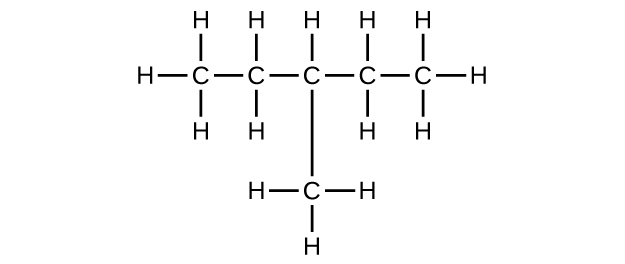(c) C6H12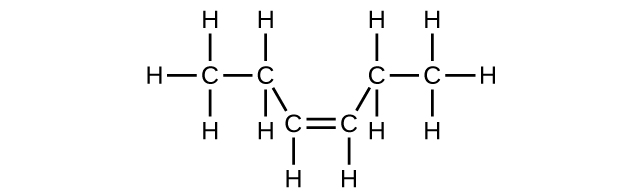(d) C6H12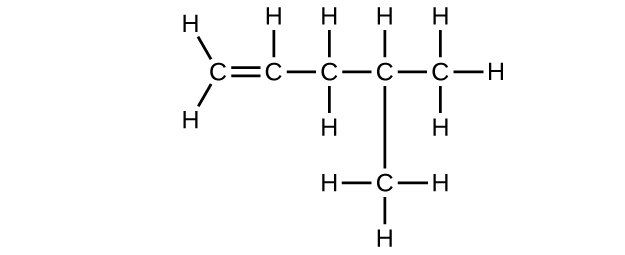(e) C6H10(f) C6H1010. Write the chemical formula, condensed formula, and Lewis structure for each of the following hydrocarbons:

1. heptane
2. 3-methylhexane
3. trans-3-heptene
4. 4-methyl-1-hexene
5. 2-heptyne
6. 3,4-dimethyl-1-pentyne

11. Give the complete IUPAC name for each of the following compounds:

1. CH3CH2CBr2CH3
2. (CH3)3CCl
3.4. $$\mathrm{CH_3CH_2C≡CH\, CH_3CH_2C≡CH}\ 5.6.7. \(\mathrm{(CH_3)_2CHCH_2CH=CH_2}$$
(a) 2,2-dibromobutane; (b) 2-chloro-2-methylpropane; (c) 2-methylbutane; (d) 1-butyne; (e) 4-fluoro-4-methyl-1-octyne; (f) trans-1-chloropropene; (g) 5-methyl-1-pentene

12. Give the complete IUPAC name for each of the following compounds:

1. (CH3)2CHF
2. CH3CHClCHClCH3
3.4. $$\mathrm{CH_3CH_2CH=CHCH_3}$$
5.6. $$\mathrm{(CH_3)_3CCH_2C≡CH}$$

13. Butane is used as a fuel in disposable lighters. Write the Lewis structure for each isomer of butane.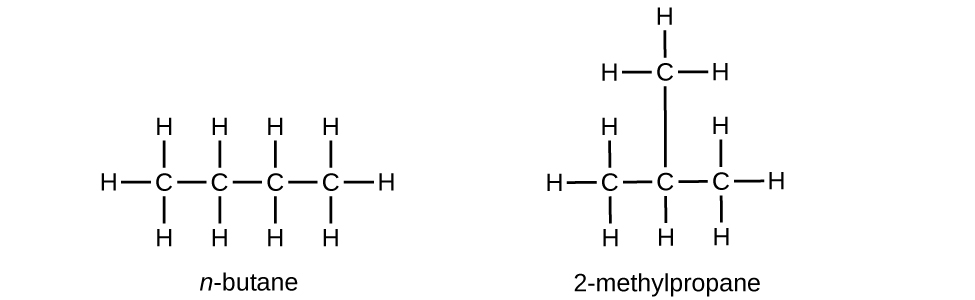14. Write Lewis structures and name the five structural isomers of hexane.

15. Write Lewis structures for the cis–trans isomers of $$\mathrm{CH_3CH=CHCl}$$.16. Write structures for the three isomers of the aromatic hydrocarbon xylene, C6H4(CH3)2.

17. Isooctane is the common name of the isomer of C8H18 used as the standard of 100 for the gasoline octane rating:1. What is the IUPAC name for the compound?
2. Name the other isomers that contain a five-carbon chain with three methyl substituents.

(a) 2,2,4-trimethylpentane; (b) 2,2,3-trimethylpentane, 2,3,4-trimethylpentane, and 2,3,3-trimethylpentane: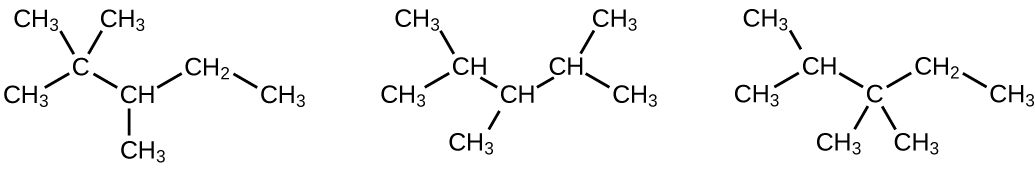18. Write Lewis structures and IUPAC names for the alkyne isomers of C4H6.

19. Write Lewis structures and IUPAC names for all isomers of C4H9Cl.20. Name and write the structures of all isomers of the propyl and butyl alkyl groups.

21. Write the structures for all the isomers of the –C5H11 alkyl group.

In the following, the carbon backbone and the appropriate number of hydrogen atoms are shown in condensed form:22. Write Lewis structures and describe the molecular geometry at each carbon atom in the following compounds:

1. cis-3-hexene
2. cis-1-chloro-2-bromoethene
3. 2-pentyne
4. trans-6-ethyl-7-methyl-2-octene

23. Benzene is one of the compounds used as an octane enhancer in unleaded gasoline. It is manufactured by the catalytic conversion of acetylene to benzene:

$\ce{3C2H2 ⟶ C6H6}$

Draw Lewis structures for these compounds, with resonance structures as appropriate, and determine the hybridization of the carbon atoms in each.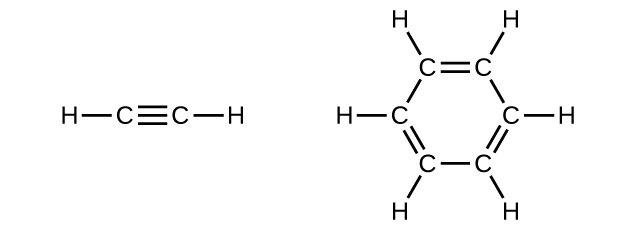In acetylene, the bonding uses sp hybrids on carbon atoms and s orbitals on hydrogen atoms. In benzene, the carbon atoms are sp2 hybridized.

24. Teflon is prepared by the polymerization of tetrafluoroethylene. Write the equation that describes the polymerization using Lewis symbols.

25. Write two complete, balanced equations for each of the following reactions, one using condensed formulas and one using Lewis structures.

1. 1 mol of 1-butyne reacts with 2 mol of iodine.
2. Pentane is burned in air.
(a) $$\mathrm{CH=CCH_2CH_3 + 2I_2⟶ CHI_2CI_2CH_2CH_3}$$(b) $$\ce{CH3CH2CH2CH2CH3 + 8O2 ⟶ 5CO2 + 6H2O}$$26. Write two complete, balanced equations for each of the following reactions, one using condensed formulas and one using Lewis structures.

1. 2-butene reacts with chlorine.
2. benzene burns in air.

27. What mass of 2-bromopropane could be prepared from 25.5 g of propene? Assume a 100% yield of product.

65.2 g

28. Acetylene is a very weak acid; however, it will react with moist silver(I) oxide and form water and a compound composed of silver and carbon. Addition of a solution of HCl to a 0.2352-g sample of the compound of silver and carbon produced acetylene and 0.2822 g of AgCl.

1. What is the empirical formula of the compound of silver and carbon?
2. The production of acetylene on addition of HCl to the compound of silver and carbon suggests that the carbon is present as the acetylide ion, $$\ce{C2^2-}$$. Write the formula of the compound showing the acetylide ion.

29. Ethylene can be produced by the pyrolysis of ethane:

$$\ce{C2H6⟶C2H4 + H2}$$

How many kilograms of ethylene is produced by the pyrolysis of 1.000 × 103 kg of ethane, assuming a 100.0% yield?